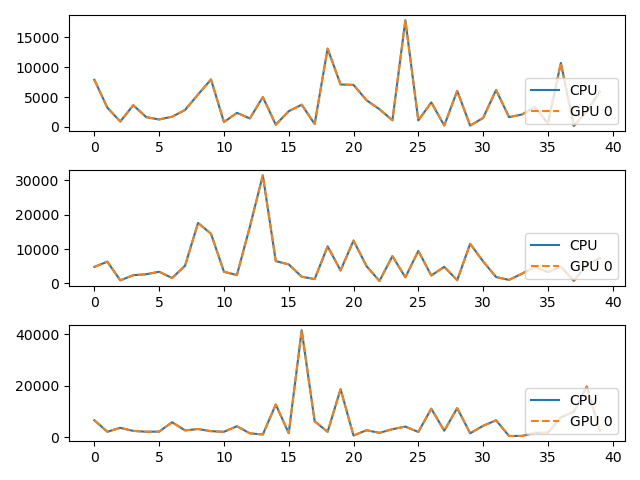# Multi GPU¶

On multi-device clusters, let’s see how to select the card on which a KeOps operation will be performed.

## Setup¶

Standard imports:

import matplotlib.pyplot as plt
import numpy as np
import GPUtil

from pykeops.numpy import Genred, Vi, Vj
from pykeops.numpy.utils import IsGpuAvailable


Define the list of gpu ids to be tested:

# By default we assume that there are two GPUs available with 0 and 1 labels:
gpuids = [0,1] if len(GPUtil.getGPUs()) > 1 else 


## KeOps Kernel using Genred¶

Define some arbitrary KeOps routine:

formula   =  'Square(p-a) * Exp(x+y)'
variables = ['x = Vi(3)','y = Vj(3)','a = Vj(1)','p = Pm(1)']

dtype = 'float32'  # May be 'float32' or 'float64'

my_routine = Genred(formula, variables, reduction_op='Sum', axis=1, dtype=dtype)


Generate some data, stored on the CPU (host) memory:

M = 1000
N = 2000
x = np.random.randn(M,3).astype(dtype)
y = np.random.randn(N,3).astype(dtype)
a = np.random.randn(N,1).astype(dtype)
p = np.random.randn(1).astype(dtype)


Launch our routine on the CPU:

c = my_routine(x, y, a, p, backend='CPU')


And on our GPUs, with copies between the Host and Device memories:

if IsGpuAvailable():
for gpuid in gpuids:
d = my_routine(x, y, a, p, backend='GPU', device_id=gpuid)
print('Relative error on gpu {}: {:1.3e}'.format( gpuid,
float( np.sum(np.abs(c - d)) / np.sum(np.abs(c)) ) ))

# Plot the results next to each other:
for i in range(3):
plt.subplot(3, 1, i+1)
plt.plot(c[:40,i],  '-', label='CPU')
plt.plot(d[:40,i], '--', label='GPU {}'.format(gpuid))
plt.legend(loc='lower right')

plt.tight_layout() ; plt.show()Out:

Relative error on gpu 0: 5.008e-07
Relative error on gpu 1: 5.008e-07
/home/bcharlier/keops/pykeops/examples/numpy/plot_gpu_select_numpy.py:74: MatplotlibDeprecationWarning:

Adding an axes using the same arguments as a previous axes currently reuses the earlier instance.  In a future version, a new instance will always be created and returned.  Meanwhile, this warning can be suppressed, and the future behavior ensured, by passing a unique label to each axes instance.

/home/bcharlier/keops/pykeops/examples/numpy/plot_gpu_select_numpy.py:74: MatplotlibDeprecationWarning:

Adding an axes using the same arguments as a previous axes currently reuses the earlier instance.  In a future version, a new instance will always be created and returned.  Meanwhile, this warning can be suppressed, and the future behavior ensured, by passing a unique label to each axes instance.

/home/bcharlier/keops/pykeops/examples/numpy/plot_gpu_select_numpy.py:74: MatplotlibDeprecationWarning:

Adding an axes using the same arguments as a previous axes currently reuses the earlier instance.  In a future version, a new instance will always be created and returned.  Meanwhile, this warning can be suppressed, and the future behavior ensured, by passing a unique label to each axes instance.


## Using LazyTensor¶

Launch our routine on the CPU:

xi, yj, aj = Vi(x), Vj(y), Vj(a)
c = ((p - aj) ** 2 * (xi + yj).exp()).sum(axis=1, backend='CPU')


And on the GPUs, with copies between the Host and Device memories:

if IsGpuAvailable():
for gpuid in gpuids:
d = ((p - aj) ** 2 * (xi + yj).exp()).sum(axis=1, backend='GPU', device_id=gpuid)
print('Relative error on gpu {}: {:1.3e}'.format(gpuid,
float(np.sum(np.abs(c - d)) / np.sum(np.abs(c)))))

# Plot the results next to each other:
for i in range(3):
plt.subplot(3, 1, i + 1)
plt.plot(c[:40, i], '-', label='CPU')
plt.plot(d[:40, i], '--', label='GPU {}'.format(gpuid))
plt.legend(loc='lower right')

plt.tight_layout();
plt.show()Out:

Relative error on gpu 0: 5.008e-07
Relative error on gpu 1: 5.008e-07
/home/bcharlier/keops/pykeops/examples/numpy/plot_gpu_select_numpy.py:104: MatplotlibDeprecationWarning:

Adding an axes using the same arguments as a previous axes currently reuses the earlier instance.  In a future version, a new instance will always be created and returned.  Meanwhile, this warning can be suppressed, and the future behavior ensured, by passing a unique label to each axes instance.

/home/bcharlier/keops/pykeops/examples/numpy/plot_gpu_select_numpy.py:104: MatplotlibDeprecationWarning:

Adding an axes using the same arguments as a previous axes currently reuses the earlier instance.  In a future version, a new instance will always be created and returned.  Meanwhile, this warning can be suppressed, and the future behavior ensured, by passing a unique label to each axes instance.

/home/bcharlier/keops/pykeops/examples/numpy/plot_gpu_select_numpy.py:104: MatplotlibDeprecationWarning:

Adding an axes using the same arguments as a previous axes currently reuses the earlier instance.  In a future version, a new instance will always be created and returned.  Meanwhile, this warning can be suppressed, and the future behavior ensured, by passing a unique label to each axes instance.


Total running time of the script: ( 0 minutes 1.201 seconds)

Gallery generated by Sphinx-Gallery